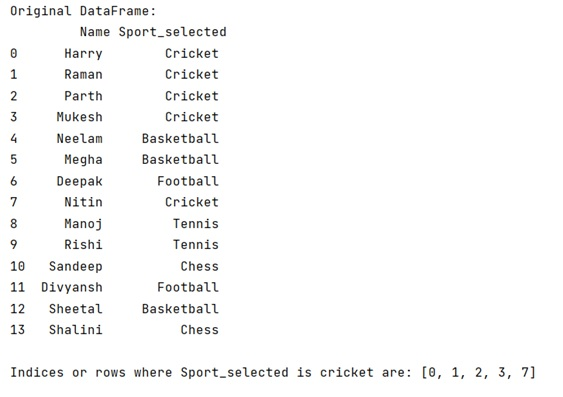# Python Pandas: Get index of rows which column matches certain value

Given a DataFrame, we have to get the index of rows which column matches certain value.
Submitted by Pranit Sharma, on April 29, 2022

DataFrame rows are based on the index values. We can manipulate both rows and columns in pandas. On the other hand, index are the integer values representing the number of rows and columns separately. We can perform many operations on rows of a DataFrame on the basis of a specific condition.

## Problem statement

Given a DataFrame, we have to get the index of rows which column matches certain value.

## Getting index of rows which column matches certain value

Following is the syntax for achieving this task:

```DataFrame.index[df['column_name']=='particular_value'].tolist()
```

Here, the above syntax will return a list of indices of rows whose column matches the specific condition passed inside the DataFrame.index() method.

Note

To work with pandas, we need to import pandas package first, below is the syntax:

```import pandas as pd
```

Let us understand with the help of an example.

## Python program to get index of Pandas rows which column matches certain value

```# Importing pandas package
import pandas as pd

# Creating a dictionary
dict = {
'Name':['Harry','Raman','Parth','Mukesh','Neelam','Megha','Deepak','Nitin','Manoj','Rishi','Sandeep','Divyansh','Sheetal','Shalini'],
}

# Creating a DataFrame
df=pd.DataFrame(dict)

# Display original DataFrame
print("Original DataFrame:\n",df,"\n")

# Generating a list if all the indices of rows
# whose column value matches a condition
indices_list = df.index[df['Sport_selected']=='Cricket'].tolist()

# Display list of indices
print("Indices or rows where Sport_selected is cricket are:",indices_list)
```

### Output

The output of the above program is: